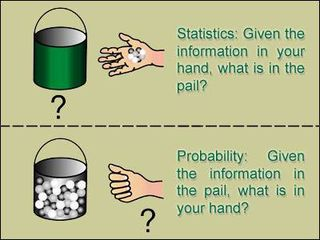## 概率空间

1. $\mathcal{\Omega}$－Sample space 样本空间，试验中所有可能结果的集合。（注：每个结果需要互斥，所有可能结果必须被穷举）
2. $\mathcal{F}$－Set of events 事件集合，是$\Omega$的一些子集构成的集合，并且它需要满足以下三点特性（也就是必须是$\sigma$－field）：
• $\mathcal{\Phi \in F}$（也就是必须包含不可能事件）
• 如果$E\in F,E^C \in \mathcal{F}$。
• 如果$E_1,E_2,\cdots ,E_i \in \mathcal{F}$,那么 $U_{i=1}^{\infty}E_i \in \mathcal{F}$
1. $\mathcal{P}$－Probability measure 概率测度（或概率），描述一次随机试验中被包含在 $\mathcal{F}$中的所有事件的可能性。并且它「碰巧」也需要满足三点特性：
• $0\leq P(E_i)\leq 1$（实际限制了总测度为1）
• $P(\Omega)=1$（包含样本空间并且概率为1）
• 如果$E_1,E_2,\cdots ,E_i$是互斥事件,那么$P\{U_{i=1}^{\infty} E_i \}=\sum_{i=1}^{\infty} E_i$

## 谈斯特林公式

$$n! \sim \sqrt{2\pi }n^{n+\frac{1}{2} }e^{-n}$$

### Stirling与概率问题

【问题1】 抛 $2n$ 次硬币，正面恰好出现 $n$ 次的概率又多大？ 根据组合学，抛 $2n$ 次硬币，共有 $2^{2n}$ 种可能，假设每一种发生的结果机会均等，那么 $2n$ 次中有 $n$ 次正面的结果共有 $C_{n}^{2n}$种，故得概率为

$p_{2n} = \frac{C_n^{2n}}{2^{2n}} = \frac{(2n)!}{2^{2n}\cdot n!\cdot n!} \qquad (1)$

【问题2】当$n$很大时，如何估算？明确地说：当$n$趋向$\infty$时，$n!$的渐进相等式（Asymptotically equal formula）是什么？

$$\lim_{n \to \infty} \frac{n!}{a_n} = 1,\quad n! \sim a_n$$

$n!$的渐进相等式（Asymptotically equal formula）存在吗？如何找？ 这时候就需要Stirling公式出场了。

### 初步否定常识性的概率

【补题】 设$\{a_n\},\{b_n\},\{c_n\}$及$\{d_n\}$皆为正项数列且 $\lim_{n \to \infty} \frac{a_n}{b_n} = l$。若 $a_n \sim c_n$且$b_n \sim d_n$，则 $\lim_{n \to \infty} \frac{c_n}{d_n} = l$。 接着计算「丢$2n$次硬币，恰好出现$n$次正面的机率$p_{2 n}$在 $n \to \infty$的极限」

$$\lim_{n \to \infty}p_{2n} = \lim_{n \to \infty} \frac{(2n)!}{2^{2n}n!n!} \\ =\lim_{n \to \infty} \frac{\sqrt{2\pi 2n}(2n)^{2n}e^{-2n}}{2^{2n}\cdot 2\pi \cdot n\cdot n^{2n}\cdot e^{-2n}} \\ =\lim_{n \to \infty}\frac{1}{\sqrt{\pi n}}=0$$

【定理3】 $\lim_{n \rightarrow \infty} p_{2n} = 0$。 因此，当 $n \to \infty$时，$p_{2n}$不但不如原先预计的趋近于1（即必然发生），反而是趋近于0（即不可能发生）。这警告我们，概率的解释与经验有很大出入。

【常识性的说法】「丢很多次硬币正面大约占一半。」如果将「大约占一半」， 解释为「恰好是一半」的说法，点估计显然是不对的。如何修正呢？自然想到的是改用区间估计的说法。

$$f(n) = \begin{cases} \epsilon_k = 1, & \text{第K次硬币得到正面 } \\ \epsilon_k = 0, & \text{第K次硬币得到反面} \end{cases}$$

$$P(n-a \leq S_{2n} \leq n+a) = \sum_{k=n-a}^{n+a} C_{k}^{2n}\cdot \frac{1}{2^{2n} } \\ \sim \frac{2a}{ \sqrt{\pi n}} \rightarrow 0 ,when \quad n \rightarrow \infty$$

【定理4】：对任意固定数$a > 0$， $$\lim_{b \to \infty} P( \mid S_{2n} - n \mid \leq a ) = 0$$

$$\frac{ C_{k}^{2n+1}\cdot \frac{1}{ 2^{2n+1} } }{C_{k}^{2n} \frac{1}{2^{2n}}} = \frac {2n+1}{2n+1-k} \cdot \frac{1}{2} \to \frac{1}{2}$$ 所以 $$P( -a \leq S_{2n+1} - \frac{2n+1}{2} \leq a ) \\ = \sum_{k=-a+ \frac {2n+1}{2}}^{a+ \frac {2n+1}{2}} C_k^{2n} \frac{1}{2^{2n+1}}\\ \sim \frac{1}{2}\sum_{k=-a+ \frac {2n+1}{2}}^{a+ \frac {2n+1}{2}} C_k^{2n} \frac{1}{2^{2n}} \to 0$$

【定理6】：对任意有限正数$a$，恒有

$$\lim_{n \to \infty} P(-a \leq S_n - \frac{n}{2} \leq a) = 0$$

1. 表面来看，Stirling 公式并不漂亮，但却很多用途，它是揭开许多深刻奥秘的钥匙。在研究二项分布的性质时，De Moivre 最先得到这个公式（1718年）；后来James Stirling 在1730年又重新得到它。
1. $n!$的连续变化就是Gamma函数，内容精彩丰富，这是Euler的贡献。

## 附录

$$log{n!}=log{1}+log{2}+\cdots+log{n}$$

$$\int_{k-1}^{k} \log{x} dx < \log{n!} < \int_{k}^{k+1}\log{x}dx$$ 把上式对$k$从$1$到$n$相加得到

$$\int_{0}^{n}\log{x}dx < \log{n!} < \int_{1}^{n+1}\log{x}dx \qquad(1)$$ $$\Leftrightarrow n\log{n}-n < \log{n!} < (n+1)\log{(n+1)}-n \qquad(2)$$ 这是一个双重不等式，提示我们把$\log {n!}$与两端数的算数平均做比较，即想到$(n+\frac{1}{2})\log{n}-n$ $$d_n=\log{n!}-(n+\frac{1}{2})\log{n}+n \qquad (3)$$ $$d_n - d_{n+1}=\log{n!}-(n+\frac{1}{2})\log\frac{n+1}{n}-1$$ $$\frac{n+1}{n}=\frac{1+\frac{1}{2n+1}}{1-\frac{1}{2n+1}}$$ $\boldsymbol{Taylor}$展开得 $$d_n - d_{n+1}=\frac{1}{3(2n+1)^2}+\frac{1}{5(2n+1)^4}+\cdots$$ 把上式与一个公比的几何级数进行比较得 $$0 < d_n-d_{n+1} < \frac{1}{3\left [ (2n+1)^2-1 \right ]}=\frac{1}{12n}-\frac{1}{12(n+1)}$$ 知是${d_n}$下降序列，说明序列${d_n-(12n)^{-1} }$是上升的，因此，极限$\lim d_n = C$ 存在且有限，但是，由$(3)$知：$d_n \rightarrow C$等价于 $$n! \sim e^C \cdot n^{n+\frac{1}{2} } \cdot e^{-n}$$